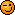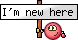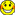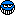Tech Log The very best in practical technical discussion on the web

Accurate Temperature Correction Formula

Join Date: Jun 2001
Location: at the edge of the alps
Posts: 325
Accurate Temperature Correction Formula

I am trying to develop an Excel formula for exact temperature correction for a friend, following the "accurate" formula from PANS-OPS Section III 4.3.4.1:

Correction = trianglehPaircraft - trianglehGaircraft = (-triangleTstd/L0) * Ln[(1 + L0 * trianglehPaircraft)/(T0 + L0*hPaerodrome)]

Where:
trianglehPaircraft = Aircraft height above aerodrome (pressure) ft
trianglehGaircraft = Aircraft height above aerodrome (geopotential) ft
triangleTstd = Temperature deviation from the ISA temperature K
L0 = Standard temperature lapse rate with pressure
altitude in the first layer (sea level to tropopause)
of the ISA.(See note (b) below) K/ft
T0 = Standard temperature at sea level K
hPaerodrome = Aerodrome height (pressure) ft

I have devised the following Excel Formula:

where "ISADEV" is the temperature deviation from ISA (usually a negative number in cold weather)
"MDH" is the minimum descent height in ft
"APAlt" is the airport altitude in ft
-0,0019812 is the lapse rate in K/ft and 288,15 is ISA/sea level in K.

This results in meaningless results.

I am challenged by not really understanding why the basic formula should work and would be grateful for any hints on where I might have erred in applying it.Join Date: May 2005
Location: France / UK
Age: 64
Posts: 899
4ft per degree C dev per 1000ft above source elevation.

I don't know how to express that in terms that would fit your excel formula, sorry!Join Date: Jun 2003
Location: LSZH, oder in der Nähe
Posts: 348
It all seems terribly overcomplicatedThe ISA model is quite simple and straightforward...

Metric:
T = T0 - 6.5 (h/1000)
where
T0 is the ISA temperature (15C)
h is altitude in meters
T is the temperature at altitude h
Imperial:
T = T0 - 1.98 (h/1000)
where
T0 is the ISA temperature (15C)
h is altitude in feet
T is the temperature at altitude hJoin Date: May 2005
Location: France / UK
Age: 64
Posts: 899
WeeJeem,

Thanks for those formulæ but I think that Alpine Flyer is looking for a formula that expresses the error between indicated and true altitude, given a deviation from the ISA temperature.Join Date: Jun 2001
Location: at the edge of the alps
Posts: 325
@eckhard: the 4% formula is the "simple" formula but limited to temperatures down to -15C. Beyond that, PANS OPS offers a table that covers lower temperatures but is limited to 5000ft and two more formulae, the more complicated of which was the reason for my post.

As probably all those formulae are but child's play for a modern Computer, IMHO it does not make sense to use the simplified versions rather than going for the most accurate calculation.

@WeeJam: I have understood lapse rate (which is 1,9812C per 1000ft to be exact) but lapse rate is just one building block for calculating cold temperature correction.

Any other input welcome. It must be possible to solve this!Join Date: Feb 2000
Location: 500 miles from Chaikhosi, Yogistan
Posts: 3,447
There's a simpler ICAO formula that's pretty good, without the ln....

Unless your friend is seriously seriously scientific (and in which case would know how to use it anyway?) try that one.Join Date: May 2006
Location: Australia
Posts: 394
Try this...

=(B6-B2)*((15-(B8+(0.00198*B2)))/((273+(B8+(0.00198*B2)))-(0.5*0.00198*((B6-B2)+B2))))

Where: B6 is aircraft alt (ft)
B2 is aerodrome elevation (ft)
B8 is aerodrome temperature

The formula is copied straight from my spreadsheet and should work if the correct values are assigned. The formula is an older one but should work for your purposes.

AlphaJoin Date: Jun 2001
Location: at the edge of the alps
Posts: 325
@alphacentauri:

Thanks, this seems to be the first equation from 4.3.3 that is valid for altimeter setting sources up to 10.000ft (which should be sufficient for Europe) but only 5000ft above source.

According to 8168 this equation was used to calculate the "standard table" that is also valid up to 5000ft above source.

So it can't be used for higher altitudes of rather low-lying airports in mountainous areas (such as Innsbruck or Geneva) which have minimum crossing altitudes more than 5000ft above the airport.Join Date: Oct 2016
Location: Japan
Posts: 1
Cannot calculate Accurate Method

Hi everyone,

I have a simple question, cannot calculate following formula for use following example value as Ln value was minus. (excel shows "#NUM!" error)
How can I get a correct answer ?

Ex.
Airport Temp; -40C
Airport Elevation; 10ft
Aircraft Altitude; 3000ft

where "ISADEV" is the temperature deviation from ISA (usually a negative number in cold weather)
"MDH" is the minimum descent height in ft
"APAlt" is the airport altitude in ft
-0,0019812 is the lapse rate in K/ft and 288,15 is ISA/sea level in K.Join Date: Jan 2019
Location: Hamburg
Posts: 2
Hi to all,
has anyone got a solution for this? Maybe there's a mistake within the displayed formula.
I've tried out everything (+/- values, °C iso K...) but there's never a result that makes any sense.
Has anyone access to the equation #24 of the ESDU publication Performance, Vol. 2, Item No. 77022?
Thanxx al lot so far...Join Date: May 2005
Location: France / UK
Age: 64
Posts: 899
Error of approx 660ft? True altitude 2340ftJoin Date: Jan 2004
Location: Hiding
Posts: 988

This might help. There's a link to a spreadsheet at the bottom of the article under "Further Reading"Join Date: Jan 2011
Location: Seattle
Posts: 618
I have devised the following Excel Formula:

where "ISADEV" is the temperature deviation from ISA (usually a negative number in cold weather)
"MDH" is the minimum descent height in ft
"APAlt" is the airport altitude in ft
-0,0019812 is the lapse rate in K/ft and 288,15 is ISA/sea level in K.

This results in meaningless results.

I am challenged by not really understanding why the basic formula should work and would be grateful for any hints on where I might have erred in applying it.
A quick examination of this formula reveals that for MDH over about 500 ft, the term (1+-0,0019812*"MDH") in your spreadsheet formula turns negative. The natural log (LN) of a negative number is undefined (unless you are an electrical engineer).Join Date: Jan 2019
Location: Hamburg
Posts: 2
Good news if anyone is interested....
I found the bug, the accompaning pdf documentation from Eurocontrol folowing the link in post #12 shows the correct equation.

the 1+ is prior the fraction... Sombody within ICAO seems not to be aware of maths basics ;-)
Like that, it works fine...

BGYou may not post new threads
You may not post replies
You may not post attachments
You may not edit your posts

BB code is On
Smilies are On
[IMG] code is On
HTML code is OffShow Printable VersionEmail this Page